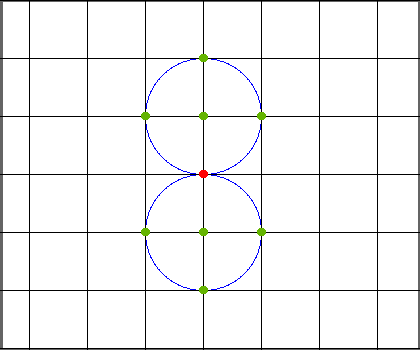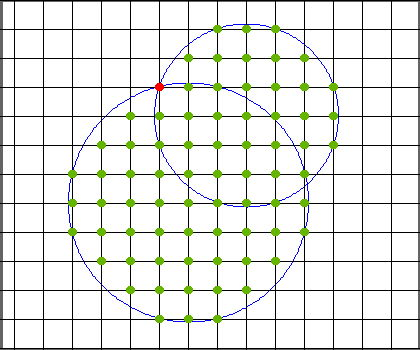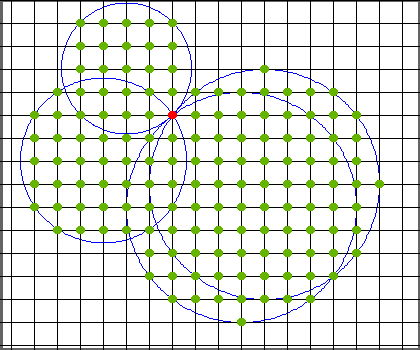시간 제한 메모리 제한 제출 정답 맞은 사람 정답 비율
1 초 256 MB 10 3 3 30.000%

## 문제

There are $N$ circles on a plane. The center of the $i$-th circle is $(x_i, y_i)$, and the radius of this circle is $\sqrt{x_i^2 + y_i^2}$.

Count the number of lattice points (points with integer coordinates) that are inside at least one of the circles. Note that the boundaries are considered to be inside.

## 입력

$N$
$x_1$ $y_1$
$x_2$ $y_2$
$\vdots$
$x_N$ $y_N$

## 출력

Print the answer in a single line.

## 제한

• $1 \leq N \leq 10^5$
• $-10^5 \leq x_i, y_i \leq 10^5$
• $(x_i, y_i)$ are pairwise distinct.
• $(x_i,y_i) \neq (0, 0)$
• All values in the input are integers.

## 예제 입력 1

2
0 1
0 -1


## 예제 출력 1

9## 예제 입력 2

2
3 1
1 4


## 예제 출력 2

74## 예제 입력 3

4
3 4
4 3
-2 -2
-3 2


## 예제 출력 3

146